## The Michelson Morley Experiment: Some Analysis

Lets discuss the Michelson Morley Experiment from two points of view. First, assuming there is an ether which picks one frame in which the velocity of light isand second, if the speed of light is the same in every frame. In the first case, we want to see if the experiment would be sensitive to the motion of the earth through the ether. In the second case we want to see what problems the constancy of the speed of light presents. A diagram of the MM apparatus is show below.Light from a single source was split with a half silvered mirror. In the rest frame of the apparatus, half the beam transited a lengthperpendicular to the motion of the earth, and the other half transit-ted a lengthparallel to the motion of the earth. If the earth is in the rest frame of the ether (which it can't be for the all the time), both beams go the same distance at the same velocity and are in phase.

We will use some of the standard symbols of special relativity in this calculation.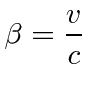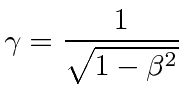If the earth is moving through the ether at a velocity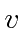, and light moves at a velocitythrough the medium of the ether, then a careful calculation is needed. In the direction parallel to the velocity of the earth through the ether, the time taken for the round trip is.trip out to mirrortrip back to detector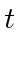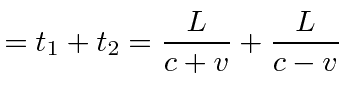round trip time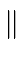toIn the direction perpendicular to the velocity of the earth through the ether, the light must actually travel at an angle.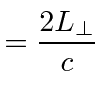time for full tripto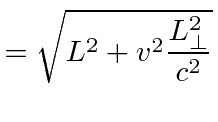distance ofpath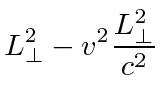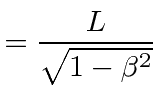round trip timetoThe difference in time would be.The MM interferometer had a length of 11m. The earth's velocity is aboutmeters per second or about 10000 times smaller than the speed of light. The distance difference seen by the interferometer will be about.This is a large fraction of the wavelength of light so it would be quite detectable.

In the case that the laws of physics and the speed of light are the same in any frame, it is certainly easiest to compute what will happen in the rest frame of the experiment. In that frame, things are very simple.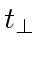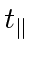The interference pattern will not move as the apparatus is rotated no matter how the earth is moving.

Lets look at it (with no relativity), for example, in the frame in which the sun is at rest, which is very much like the putative ether frame. Light moves at the speed of light in that frame and the calculation we had for the ether is correct, if we assume that the lengths are the same in that frame.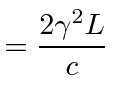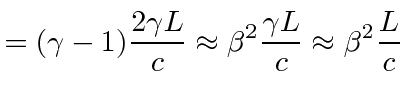It is not consistent with the calculation in the rest frame. Fitzgerald postulated that the length along the direction of motion changes as we transform to a moving frame.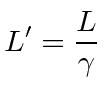This would make the interference perfect again. We will show in the next section that this is true and is known as Lorentz contraction of lengths.

Jim Branson 2012-10-21